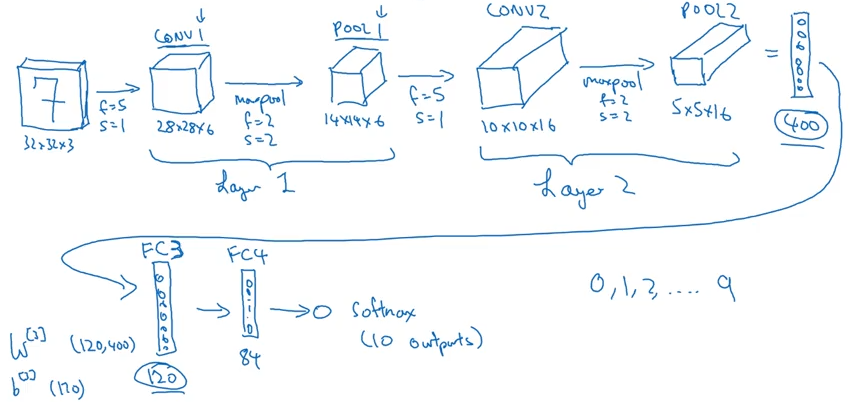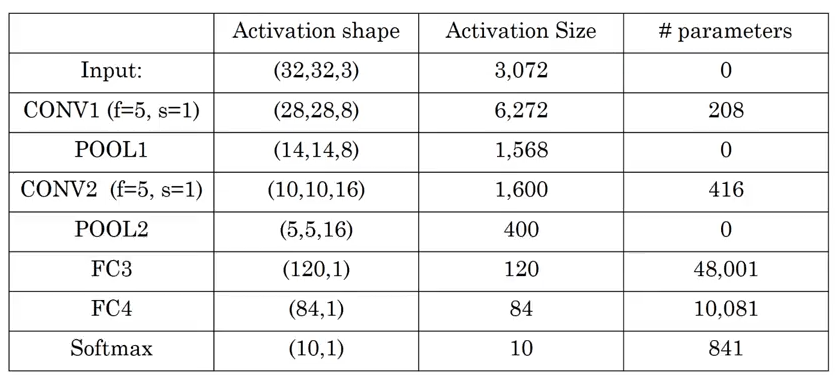# CNN organization

Now that we’ve got a few notebooks outlining the intuition and mechanics of Convolution with image-based Neural Nets, let’s talk about how we go about structuring our model.

### Example Architecture

Andrew Ng maps out a potential architecture approach for modeling an MNist classifier.

from IPython.display import Image

Image('images/conv_mnist.png')Few things to note here:

• Because there aren’t any learned parameters in our Pooling layers, we consider them “in the same layer as the adjacent Convolution step”
• The hyperparameters numFilters, filterShape, filterStride were all arbitrarily chosen and can be modified/explored from layer to layer to try and increase performance
• We do two layers of Convolution/pooling and then start using the same dense, robust layers as we’ve seen with our usual Networks.
• We could have also used more Convolutional layers or more Dense layers– in fact most CV applications do

### Breaking Down the Numbers

He then goes on to write out the shape, size, and number of parameters for each layer (where ‘size’ is the number of distinct elements in the matrix)

Image('images/conv_mnist_metadata.png')Generally speaking, the following typically hold true:

• The Activation Size decreases over the course of the network
• The number of learned/trained parameters is dramatically higher in the dense layers than the convolutional layers